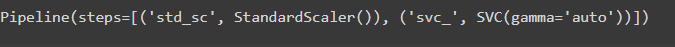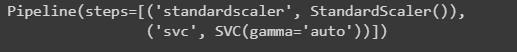GFG App
Open AppBrowser
Continue

# What is the difference between pipeline and make_pipeline in scikit?

Generally, a machine learning pipeline is a series of steps, executed in an order wise to automate the machine learning workflows. A series of steps include training, splitting, and deploying the model.

## Pipeline

It is used to execute the process sequentially and execute the steps, transformers, or estimators are named manually. Transformers and estimators are the parameters to fit the model and tune for its model accuracy.

Syntax: class sklearn.pipeline.Pipeline(steps, *, memory=None, verbose=False)

Note: In the above syntax, steps(can be represented as an array) are represented as a sequence of functions to be executed in completing in the task.

Example:

Here we are going to create a pipeline using Pipeline() methods.

## Python3

 `# import required modules ` `import` `numpy as np ` `from` `sklearn.svm ``import` `SVC ` `from` `sklearn.preprocessing ``import` `StandardScaler ` `from` `sklearn.pipeline ``import` `Pipeline ` ` `  `# declare X, used as a feature with  ` `# nested array ` `X ``=` `np.array([[``1``, ``2``], [``3``, ``4``], [``5``, ``6``], [``7``, ``8``]]) ` ` `  `# declare y as target variable ` `y ``=` `np.array([``9``, ``10``, ``11``, ``12``]) ` ` `  `# pipeline is created using Pipeline() ` `pipe ``=` `Pipeline([(``'std_sc'``, StandardScaler()), ` `                 ``(``'svc_'``, SVC(gamma``=``'auto'``))]) ` `pipe.fit(X, y) `

Output:## make_pipeline

make_pipleine is an advanced method in scikit learn, in which the naming of the estimators or transformers are done automatically.

Syntax: sklearn.pipeline.make_pipeline(*steps, memory=None, verbose=False)

Example:

Here we are going to make pipeline using make_pipeline() methods.

## Python3

 `# import required modules ` `import` `numpy as np ` `from` `sklearn.pipeline ``import` `make_pipeline ` `from` `sklearn.preprocessing ``import` `StandardScaler ` `from` `sklearn.svm ``import` `SVC ` ` `  `# declare X, used as a feature with  ` `# nested array ` `X ``=` `np.array([[``1``, ``2``], [``3``, ``4``], [``5``, ``6``], [``7``, ``8``]]) ` ` `  `# declare y as target variable ` `y ``=` `np.array([``9``, ``10``, ``11``, ``12``]) ` ` `  `# pipeline is created using make_pipeline() ` `mp ``=` `make_pipeline(StandardScaler(), ` `                   ``SVC(gamma``=``'auto'``)) ` `mp.fit(X, y) `

Output:## Table of difference between pipeline and make_pipeline in scikit

My Personal Notes arrow_drop_up